# 4.2 Basis Functions and Matrix Representation

For the purpose of discretization one can expand the HAMILTONian, self-energies, and GREEN's functions in terms of some basis functions to obtain the corresponding matrices. In the tight-binding method one can take the basis functions to be any set of localized functions, such as atomic s- and p-orbitals [116,122], WANNIER functions , and so forth. A common approximation used to describe the HAMILTONian of layered structures consists of non-vanishing interactions only between nearest neighbor layers. That is, each layer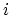interacts only with itself and its nearest neighbor layers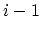and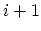. Then the single particle HAMILTONian of the layered structure is a block tri-diagonal matrix, where diagonal blocks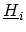represent the Hamiltonian of layerand off-diagonal blocks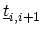represent interaction between layersand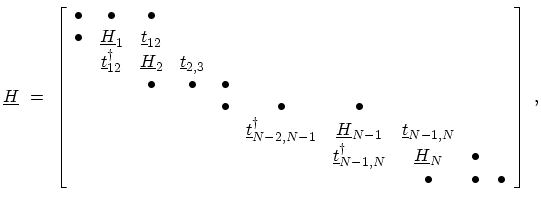(4.10)

where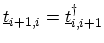. The matrix representation of the kinetic equations (3.71) and (3.72) are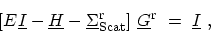(4.11)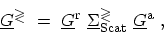(4.12)

where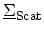is the self-energy due to scattering processes and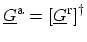.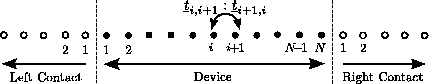One can partition the layered structure into left contact with index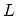, device region with index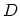, and right contact with index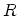(Fig. 4.5). The device corresponds to the region where one solves the transport equations and the contacts are the highly conducting regions connected to the device. While the device region consists of only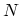layers, the matrix equation corresponding to (4.11) is infinitely dimensional due to the semi-infinite contacts. As shown in Appendix G the influence of the semi-infinite contacts can be folded into the device region by adding a self-energy to the device region. This can be viewed as an additional self-energy due to the transitions between the device and the contacts. In the next sections the matrix representation of the HAMILTONian and self-energies are discussed in detail.

M. Pourfath: Numerical Study of Quantum Transport in Carbon Nanotube-Based Transistors